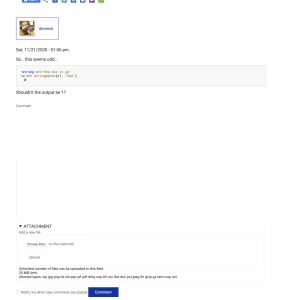# Problems entering commentsWhen I reply to a topic, if the text is greater than 5 lines, the editor goes wonky deleting entered text and show a broken text entry field.

AndyOur "web guy" was on the job this weekend. This is the first line

line 2

line 3

line 4

line 5

another line

yet another line

Here's some Igor code for testing

Function WM_MakeDataForLissajous(numDataPoints)
Variable numDataPoints

Make /O/N=(numDataPoints,3) Lissajous3D
Make/O/N=(numDataPoints,4) lissColorWave
Variable factor=2*pi/numDataPoints
Variable f1=1,f2=2,f3=3
Variable p1=0,p2=pi/4,p3=pi/2

f1 *= factor
f2 *= factor
f3 *= factor

Lissajous3D[] = sin(f1*p+p1)
Lissajous3D[] = sin(f2*p+p2)
Lissajous3D[] = sin(f3*p+p3)

lissColorWave[]=1
lissColorWave[]=(1+Lissajous3D[p] )/2
lissColorWave[]=(1+Lissajous3D[p] )/2
lissColorWave[]=(1+Lissajous3D[p] )/2
End